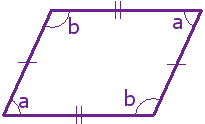# Java Program To Find Area of Parallelogram – Programs

Java program to calculate the area of a parallelogram. There may be so many ways to represent the code in order to calculate the area of a parallelogram. Here, we tried to write in just three to four lines of code.

# Scroll down for online compiler and execution tool #

If you were new to java programming and have you no idea how this following java code works about to calculate or to find the area, make sure to read out the complete article just after this code, we explained each and every line of code.

Just a couple of lines for newbies about what is a parallelogram and what are the functions of a parallelogram.

1) What is a parallelogram?

A parallelogram is a 4 shaped four-sided flat shaped with opposite sides are parallel and equal in length and opposite angles are same as well. Check it out the below figure, you will get an idea.image source

Examples: Rhombus, rectangle, square are all parallelograms.

2)  Math formula to calculate the area of a parallelogram??

The math formula to calculate the area of a parallelogram is as follows :

Height X Breadth ( B x H ), The same formula is a used in the following java program to calculate or to find the area of a parallelogram. check it out.

Consider this as Example method – 1, sample method -2 as follows :

Output :

How simple it was right? Anyways, that’s not the only way to calculate the area of a parallelogram. There may be so many different ways you can code, but the simple is better and easy to understand for newbies. The following lines will explain how this program works.

Explanation for the above code :

1)

–  Import and read the values from the given program. Whatever shit it is , you must write before starting the program.

2)

– As usual , just allocating the name for the following program. You can write whatever it is.

3)

– And the main function goes here, and the program starts executing from this panel.

4)

– Read the inputs from the system , export the data from java.util ( main head function )

5)

– Output , enter the value of the height. same as follows for the ” Breadth “.

6)

– Allocating some space in order to store the value for a particular number to execute in the next level of the program , same as follows for the next line of code.

7)

– The formula to calculate , the area of a parallelogram.

8)

– output displayed here.

Sample Method -2 # to calculate the area of a parallelogram # compiler & Execution #

There may be little bit of difference from the below code when compared with the above program, if you were closely look in the program you can understand, that we are used the ” parse “ method here but not nextdouble(); The major difference here as follows, whereas nextdouble(); can convert the ” Character ” into number from the inbuilt package java.util.

NOTE : Enter the arguments as follows ” 1-9(Height) Space 1-9(Breadth),  args and args as follows.

Whereas in the online compiler, there is no such extension exists in order to retrieve the package from util.scanner, for that purpose we are using the ” Parse “ with the help of arguments directly display on the screen. Make sure to enter the arguments for compiling and execution. If you have any doubts related to this section do comment at the end of the post.

Q. The difference between ” Int ” and “Double”? Why are we using the ” Double function here?

A > The difference is very slight, The function of ” Int ” is to read and display the I/O values just before the decimal point. The values after the decimal point will won’t display. But, in double, the values will be displayed even after the decimal point as like ” 158.22 “.

Q. Why we used ” Double”, but not ” Float”?

A > The float can store the values up to 4-Bytes, whereas ” Double ” can store the values up to 8-Bytes, you can use either of them.

# Java program to calculate the area of a parallelogram end #

x

## X Star Pattern Java Program – Patterns

Java program to print X star pattern program – We have written the below print/draw ...